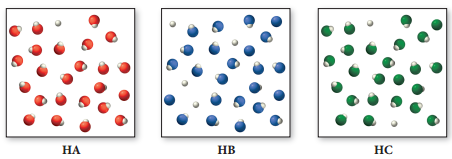# Problem: Consider the three generic weak acids HA, HB, and HC. The images shown here represent the ionization of each acid at room temperature.Which acid has the largest Ka?

###### FREE Expert Solution

Ka expression for weak acid, HA:

$\overline{){{\mathbf{K}}}_{{\mathbf{a}}}{\mathbf{=}}\frac{\mathbf{products}}{\mathbf{reactants}}{\mathbf{=}}\frac{\mathbf{\left[}{\mathbf{H}}_{\mathbf{3}}{\mathbf{O}}^{\mathbf{+}}\mathbf{\right]}\mathbf{\left[}{\mathbf{A}}^{\mathbf{-}}\mathbf{\right]}}{\mathbf{\left[}\mathbf{HA}\mathbf{\right]}}}$

**H3O+= H+

Higher Ka  Higher [H3O+] or Higher [H+]

81% (138 ratings)###### Problem Details
Consider the three generic weak acids HA, HB, and HC. The images shown here represent the ionization of each acid at room temperature.Which acid has the largest Ka?